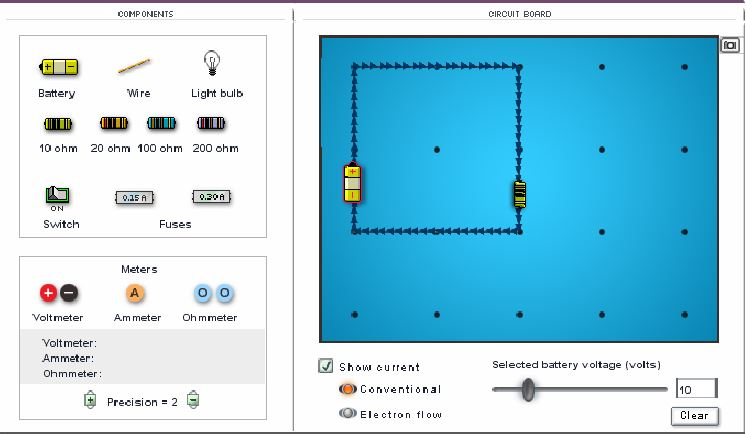# DC Electronics Exploration - Day 2

## Objective

Students will consolidate their learning about Ohm's law.

#### Big Idea

Ohm’s law relates current, voltage, and resistance in a very simple formula: V = IR.

## Overview

As we continue our exploration of electronics, students need the concepts of voltage and current to be defined. Most of electronics is simultaneously familiar (everyone buys batteries and is aware of words like "amps" and "volts") and abstract (what, exactly, are we talking about when we use those words?) Today, my goal is to make those terms more physically meaningful to students. My greater purpose is to develop a true sense of the energy transfer that happens in electric circuits as I build toward  the achievement of NGSS Performance Expectations HS-PS3-1 and HS-PS3-3 (computing energy transformations and designing devices that demonstrate the transfer of energy).

Furthermore, today's lesson is a bit abbreviated. Weather and field trips can be uncontrollable elements. Today, my classes are affected by both, forcing me to scale back on my original goals for the day.

## Defining Voltage and Current

25 minutes

Coming into this lesson, students have used the Gizmo simulation ("Circuits") to collect current and voltage data for two different resistors. They have explored circuits that look like the one below (note the measurement tools that are available in the lower left-hand corner of the screen):Before going any further, however, students need the concepts of voltage and current to be defined. I take the opportunity during this segment of class to provide some insight into these terms. The mode is very teacher-driven, with students taking notes and asking or responding to questions.

First, I want to validate students' findings about the first activity in the self-guided exploration: the induction of Ohm's Law. I show a set of axes with two sets of data representing the kinds of data students found in the activity. In that activity, students were prompted to alter the voltage, then measure the resulting current. Students, naturally then, show the y-axis as current ("I") and the x-axis as voltage ("V"). I choose the same orientation for this part of the lesson.

I spend a few minutes identifying the linear nature of this relationship ("I = m V") and ask students what they found the slope ("m") to be. Once we get clarity (see the reflection associated with this lesson for some thoughts about student thinking and questioning techniques) about the slope being 1/R, we can quickly re-write the relationship as the famous Ohm's Law. Here's a sample student graph based on a 10-ohm resistor (note the slope of 0.1!!):Next, I want to make sure that current and voltage move from abstract, mathematical ideas to more physically concrete ones. To begin, I show a simple circuit with a light bulb and focus on the idea that electrons are moving in the circuit. Students are comfortable with that idea, having seen arrows moving in the simulation. What is new is the unit of "coulombs/second," which prompts me to define the charge of the electron and to discuss the number of electrons that would make up a single coulomb of charge (close to 10 million trillion!) I use the "e" on the SmartBoard as a sample electron and move it around the circuit to simulate the motion of a single charge. Only after I feel students have a good sense of the physical nature of "coulombs per second," I introduce the more common unit: amps.

Finally, I use the same circuit to build off of the sense that students have that voltage is equivalent to energy. Batteries are, after all, a source of power. I affirm this understanding but introduce a minor variation: a volt is a joule of energy delivered to a coulomb of charge. Having talked about how many electrons are in a coulomb, students are ready to think along with me: if electrons were grouped into collections large enough to be a single coulomb, then a 9-volt battery would deliver 9 joules of energy to that group. I use the red circle on the SmartBoard to simulate a coulomb's worth of charge. I move it around the circuit, while describing the energy it gains (from the battery) and delivers (to the light bulb).

By adopting this fiction (electrons moving in collections called coulombs), we can more easily see the conservation of energy at play. A natural consequence of this thinking is that students can readily suggest that, after some time, the battery will need to be replaced as its energy has been transferred to electrons over time.

## Self-Guided Exploration of Simple Circuits

35 minutes

Students return to their self-paced exploration of circuits. Though this activity is self-paced, students today are generally moving from the induction of Ohm's Law to examining Voltage Divider and Current Divider circuits. By allowing this exploration to be self-paced, those less familiar with circuit elements can take some additional time to build their intuition without holding back others with greater familiarity with circuit ideas.

Computers are available for use. I move around the room, helping students decode instructions where necessary. A common example of misunderstanding directions is this: in Activity C, students are directed to remove the battery from the circuit in order to make a resistance measurement. Students frequently replace the battery with a wire and their ohm-meter measures zero ohms. A quick intervention on my part can reduce frustration and prevent students from developing misunderstandings about resistance.

Students continue with this work though to the end of the class. In the next lesson, we try to consolidate their learning about voltage and current divider circuits.